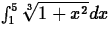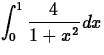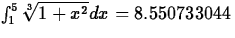## Exercises

1.
Approximatewith the Trapezoidal Rule and Simpson's Rule using 8 subintervals.
2.
Approximate, use the Trapezoidal Rule and Simpson's Rule using.
3.
For the following integralfind the minimum number of subintervals to ensure an error less than or equal to 0.01 for the following approximations.
(a)
right rectangular
(b)
trapezoid
(c)
Simpson
4.
Repeat the same process as in question 3, but now the error should be less than or equal to 0.001.
5.
Which method is more efficient and why?

Christine M Palmer
3/20/1998